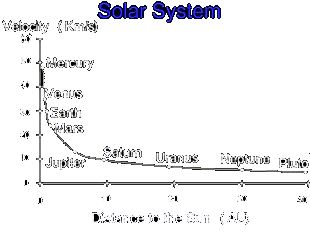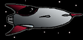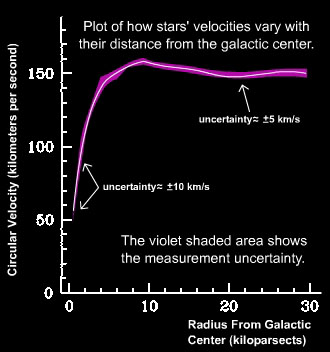### The Case for Dark Matter

##### Bizarre Rotational Velocities

What if our solar system behaved like a galaxy?

All of the planets would have the same tangential velocity. That is, they would cover the same distance in the same amount of time. However, the bodies in the outer orbits (such as Neptune) would have slower angular velocities; it would still take them longer to make a complete revolution around the sun.

In reality, our solar system does not behave like this. The outer planets have a much slower tangential speed than the inner ones.The velocity of a planet is inversely proportional to its distance from the sun.

As discussed in the Fundamentals: Gravity section we know that the gravitational force between two objects has an inverse square relationship with the distance between them. As such, Mercury experiences a greater gravitational force from the Sun than any other planet. Therefore, it's reasonable to expect Mercury to have the fastest tangential velocity in its orbit. This idea does not, however, extend to calculations of velocity of objects in clusters and galaxies; the predictions simply do not fit the observations.Sidequest: Rotational Velocity of the Solar SystemThe tangential velocity of a star is generally independent of its radial distance from Galactic Center.

When astronomers observe the rotational velocity of galaxies and clusters, they collect data which show measurements of objects at various distances from the obital center. Incidentally, in galaxies, stars' radial distances do not effect their tangential velocity around the Galactic Center. The Graph above shows a plot of this observation.

Since the prediction (stars further out should have lower tangential velocities) is inconsistent with observations (velocity is largely independent of distance), astronomers have proposed a theoretical matter, so-called "Dark Matter," to account for the results.The theory is that the dark matter is uniformly distributed, like a halo around the perimeter of the galaxy. The gravitational force resulting from the presence of this DM is what disrupts the inverse square relationship between stars' tangential velocity and their distances from the galactic center.